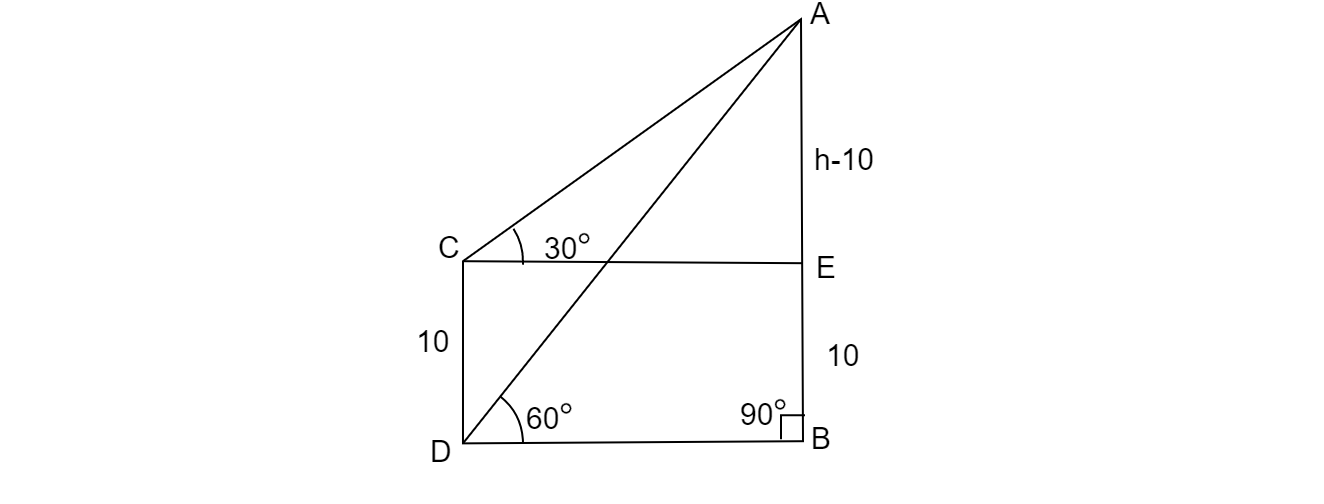# The angle of the elevation of the top of vertical tower from a point on the ground is 60°. From another point 10 m vertically above the first, its angle of elevation is 30°. Find the height of the tower.

Given: Angles of elevations $60^{o}$ and $30^{o}$ from the ground and 10 m above the ground respectively.

To do: To find the height of the tower.

Solution:
Let us say AB is the tower as shown in the fig. and $\angle ACB=60^{o}$ and $\angle ADE=30^{o}$Let the height of the tower is $h$ meter.

And given$\ CD=BE=10\ m$

In ΔABC,$\ tan60^{o} =\frac{AB}{BC} =\frac{h}{BC} =\sqrt{3}$                 $( \because \ tan60^{o} =\sqrt{3})$

or $BC=\frac{h}{\sqrt{3}}$

In ΔADE, $tan30^{o} =\frac{AE}{DE} =\frac{h-10}{BC}$                          $\left( \because \ tan30^{o} =\frac{1}{\sqrt{3}}\right) \$

$=\frac{( h-10)}{\frac{h}{\sqrt{3}}}$

$\Rightarrow \frac{\sqrt{3} \ ( h-10)}{h} =\frac{1}{\sqrt{3}}$

$\Rightarrow \ 3( h-10) =h$

$\Rightarrow 3h-30=h$

$\Rightarrow 2h=30$

$\Rightarrow h=15\ m$

$\therefore$ Height of the tower is $15\ m$.

Updated on: 10-Oct-2022

449 Views